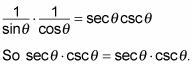##### Trigonometry Workbook For DummiesWhen asked to prove an identity, if you see a negative of a variable inside a trig function, you automatically use an even/odd identity. You first replace all trig functions with a negative variable inside the parentheses with the correct trig function using a positive variable by making use of the even/odd identities. Then you simplify the trig expression to make one side look like the other side. Here’s just one example of how this works.

With the following steps, prove this identity: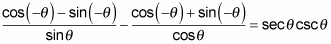1. Working with the left side, replace all negative angles and their trig functions with the even/odd identity that matches.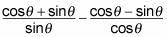2. Simplify the new expression.

Because the right side doesn’t have any fractions in it, eliminating the fractions from the left side is an excellent place to start. In order to subtract fractions, you first must find a common denominator. However, before doing that, observe that the first fraction can be split up into the sum of two fractions, as can the second fraction. By doing this step first, certain terms simplify and make your job much easier when the time comes to work with the fractions.

Therefore, you get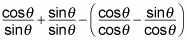which quickly simplifies to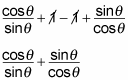Now you must find a common denominator. For this example, the common denominator is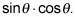Multiplying the first term by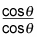and the second term by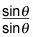gives you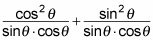You can rewrite this equation as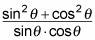Here is a Pythagorean identity in its finest form!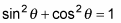is the most frequently used of the Pythagorean identities. This equation then simplifies to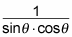Using the reciprocal identities, you get